## How to Calculate and Solve for Resistance of a Temperature Detector | Temperature Measuring Instruments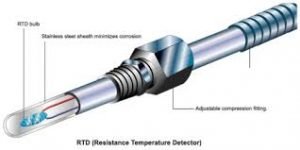The image above represents resistance of a temperature detector.

To compute for resistance of a temperature detector, four essential parameters are needed and these parameters are Reference Resistance (Ro), Temperature Co-efficient of Resistivity (α), Temperature of Thermistor (T) and Ambient Temperature (To).

The formula for calculating resistance of a temperature detector:

R = Ro [1 + α(T – To)]

Where:

R = Resistance
Ro = Reference Resistance
α = Temperature Co-efficient of Resistivity
T = Temperature of Thermistor
To = Ambient Temperature

Let’s solve an example:
Find the resistance when the reference resistance is 11, the temperature co-efficient of resistivity is 13, the temperature of thermistor is 17 and the ambient temperature is 19.

This implies that;

Ro = Reference Resistance = 11
α = Temperature Co-efficient of Resistivity = 13
T = Temperature of Thermistor = 17
To = Ambient Temperature = 19

R = Ro [1 + α(T – To)]
R = 11 x [1 + 13 x (17 – 19)]
R = 11 x [1 + 13 x (-2)]
R = 11 x [1 + -26]
R = 11 x -25
R = – 275

Therefore, the resistance of temperature detector is – 275 Ω.

## How to Calculate and Solve for Relationship between Resistance and Thermistor Temperature | Temperature Measuring Instruments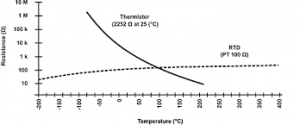The image above represents the relationship between resistance and thermistor temperature.

To compute for relationship between resistance and thermistor temperature, four essential parameters are needed and these parameters are Reference Resistance (Ro), Temperature Parameter (β), Temperature of Thermistor (T) and Ambient Temperature (To).

The formula for calculating relationship between resistance and thermistor temperature:

R = Ro eβ(1 / T – 1 / To)

Where:

R = Resistance
Ro = Reference Resistance
β = Temperature Parameter
T = Temperature of Thermistor
To = Ambient Temperature

Let’s solve an example;
Find the resistance when the reference resistance is 21, the temperature parameter is 10, the temperature of thermistor is 14 and the ambient temperature is 8.

This implies that’

Ro = Reference Resistance = 21
β = Temperature Parameter = 10
T = Temperature of Thermistor = 14
To = Ambient Temperature = 8

R = Ro eβ(1 / T – 1 / To)
R = 21 x e10 x (1 / 14 – 1 / 21)
R = 21 x e10 x (0.071 – 0.125)
R = 21 x e10 x -0.0535
R = 21 x e-0.535
R = 21 x 0.585
R = 12.29

Therefore, the relationship between resistance and thermistor temperature is 12.29 Ω.

## How to Calculate and Solve for Resistance Change of Thermistor as a First Order Approximation | Temperature Measuring Instruments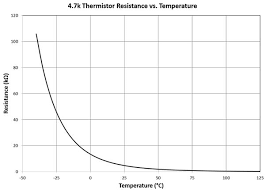The image above represents resistance change of thermistor as a first order approximation.

To compute for resistance change of thermistor as a first order approximation, three essential parameters are needed and these parameters are Value (K), Temperature of Thermistor (T) and Ambient Temperature (To).

The formula for calculating resistance change of thermistor as a first order approximation:

ΔR = k(T – To)

Where:

ΔR = Resistance Change in Thermistor as a First Order Approximation
K = Value
T = Temperature of Thermistor
To = Ambient Temperature

Let’s solve an example;
Find the resistance change in thermistor as a first order approximation when the value is 22, the temperature of thermistor is 16 and the ambient temperature is 11.

This implies that;

K = Value = 22
T = Temperature of Thermistor = 16
To = Ambient Temperature = 11

ΔR = k(T – To)
ΔR = 22 x (16 – 11)
ΔR = 22 x 5
ΔR = 110

Therefore, the resistance change of thermistor as a first order approximation is 110 Ω.

Calculating for the Value when the Resistance Change of Thermistor as a First Order Approximation, the Temperature of Thermistor and the Ambient Temperature is Given.

K = ΔR / (T – To)

Where;

K = Value
ΔR = Resistance Change in Thermistor as a First Order Approximation
T = Temperature of Thermistor
To = Ambient Temperature

Let’s solve an example;
Find the value when the resistance change in thermistor as a first order approximation is 22, the temperature of thermistor is 18 and the ambient temperature is 14.

This implies that;

ΔR = Resistance Change in Thermistor as a First Order Approximation = 22
T = Temperature of Thermistor = 18
To = Ambient Temperature = 14

K = ΔR / (T – To)
K = 22 / (18 – 14)
K = 22 / 4
K = 5.5

Therefore, the value is 5.5.

## How to Calculate and Solve for Full Bridge Output Voltage of Circuit under Strained Condition | Electrical Strain Guages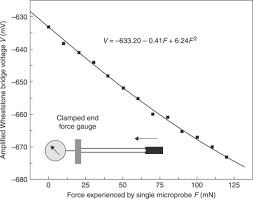The image above represents full bridge output voltage of circuit under strained condition.

To compute for full bridge output voltage of circuit under strained condition, three essential parameters are needed and these parameters are Gauge Factor (GF), Strain (ε) and Input Supply Voltage (vi).

The formula for calculating full bridge output voltage of circuit under strained condition:

vo = GF ε vi

Where:

vo = Full Bridge Circuit Output Voltage
GF = Gauge Factor
ε = Strain
vi = Input Supply Voltage

Let’s solve an example;
Find the full bridge output voltage of circuit when the guage factor is 7, the strain is 4 and the input supply voltage is 3.

This implies that;

GF = Gauge Factor = 7
ε = Strain = 4
vi = Input Supply Voltage = 3

vo = GF ε vi
vo = 7 x 4 x 3
vo = 84

Therefore, the full bridge output voltage of circuit is 84 V.

Calculating for Guage Factor when the Full Bridge Output Voltage of Circuit, the Strain and the Input Supply Voltage is Given.

GF = vo / ε . vi

Where;

GF = Gauge Factor
vo = Full Bridge Circuit Output Voltage
ε = Strain
vi = Input Supply Voltage

Let’s solve an example;
Find the guage factor when the full bridge output voltage of circuit is 10, the strain is 5 and the input supply voltage is 8.

This implies that;

vo = Full Bridge Circuit Output Voltage =10
ε = Strain =  5
vi = Input Supply Voltage = 8

GF = vo / ε . vi
GF = 105 . 8
GF = 10 / 40
GF = 0.25

Therefore, the guage factor is 0.25.

## How to Calculate and Solve for Half Bridge Output Voltage of Circuit under Strained Condition | Electrical Strain Guages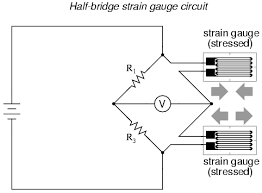The image above represents half bridge output voltage of circuit under strained condition.

To compute for half bridge output voltage of circuit under strained condition, three essential parameters are needed and these parameters are Gauge Factor (GF), Strain (ε) and Input Supply Voltage (vi).

The formula for calculating half bridge output voltage of circuit under strained condition:

vo = (GF2) . ε vi

Where:

vo = Half Bridge Circuit Output Voltage
GF = Gauge Factor
ε = Strain
vi = Input Supply Voltage

Let’s solve an example;
Find the half bridge output voltage of circuit when the guage factor is 8, the strain is 4 and the input supply voltage is 2.

This implies that;

GF = Gauge Factor = 8
ε = Strain = 4
vi = Input Supply Voltage = 2

vo = (GF2) . ε vi
vo82 x 4 x 2
vo = 4 x 4 x 2
vo = 32

Therefore, the half bridge output voltage of circuit is 32 V.

Calculating for Guage Factor when the Half Bridge Output Voltage of Circuit, the Strain and the Input Supply Voltage is Given.

GF = vo x 2 / ε . vi

Where;

GF = Gauge Factor
vo = Half Bridge Circuit Output Voltage
ε = Strain
vi = Input Supply Voltage

Let’s solve an example;
Find the guage factor when the half bridge output voltage of circuit is 10, the strain is 15 and the input supply voltage is 18.

This implies that;

vo = Half Bridge Circuit Output Voltage =10
ε = Strain =  15
vi = Input Supply Voltage = 18

GF = vo x 2 / ε . vi
GF = 10 x 2 / 15 . 18
GF20 / 270
GF = 0.074

Therefore, the guage factor is 0.074.

## How to Calculate and Solve for Quarter Bridge Output Voltage of Circuit under Strained Condition | Electrical Strain Guages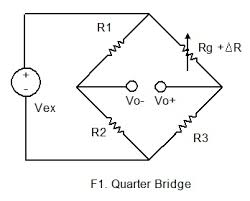The image above represents quarter bridge output voltage of circuit under strained condition.

To compute for quarter bridge output voltage of circuit under strained condition, three essential parameters are needed and these parameters are Gauge Factor (GF), Strain (ε) and Input Supply Voltage (vi).

The formula for calculating quarter bridge output voltage of circuit under strained condition:

vo = (GF / 4) . ε vi

Where:

vo = Quarter Bridge Circuit Output Voltage
GF = Gauge Factor
ε = Strain
vi = Input Supply Voltage

Let’s solve an example;
Find the quarter bridge output voltage of circuit when the guage factor is 18, the strain is 16 and the input supply voltage is 12.

This implies that;

GF = Gauge Factor = 18
ε = Strain = 16
vi = Input Supply Voltage = 12

vo = (GF / 4) . ε vi
vo = 18 / 4 x 16 x 12
vo = 4.5 x 16 x 12
vo = 864

Therefore, the quarter bridge output voltage of circuit is 864 V.

Calculating for Guage Factor when the Quarter Bridge Output Voltage of Circuit, the Strain and the Input Supply Voltage is Given.

GF = vo x 4 / ε . vi

Where;

GF = Gauge Factor
vo = Quarter Bridge Circuit Output Voltage
ε = Strain
vi = Input Supply Voltage

Let’s solve an example;
Find the guage factor when the quarter bridge output voltage of circuit is 10, the strain is 15 and the input supply voltage is 18.

This implies that;

vo = Quarter Bridge Circuit Output Voltage =10
ε = Strain =  15
vi = Input Supply Voltage = 18

GF = vo x 4 / ε . vi
GF = 10 x 4 / 15 . 18
GF = 40 / 270
GF = 0.148

Therefore, the guage factor is 0.148.

## How to Calculate and Solve for Ballast Output Voltage of Circuit under Strained Condition | Electrical Strain Guages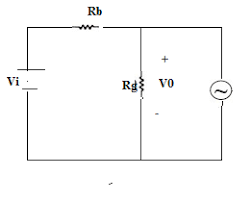The image above represents ballast output voltage of circuit under strained condition.

To compute for ballast output voltage of circuit under strained condition, three essential parameters are needed and these parameters are Gauge Factor (GF), Strain (ε) and Input Supply Voltage (vi).

The formula for calculating ballast output voltage of circuit under strained condition:

vo = (GF / 4) . ε vi

Where:

vo = Ballast Circuit Output Voltage
GF = Gauge Factor
ε = Strain
vi = Input Supply Voltage

Let’s solve an example;
Find the ballast output voltage of circuit when the guage factor is 14, the strain is 12 and the input supply voltage is 10.

This implies that;

GF = Gauge Factor = 14
ε = Strain = 12
vi = Input Supply Voltage = 10

vo = (GF / 4) . ε vi
vo = 14 / 4 x 12 x 10
vo = 3.5 x 12 x 10
vo = 420

Therefore, the ballast output voltage of circuit is 420 V.

Calculating for Guage Factor when the Ballast Output Voltage of Circuit, the Strain and the Input Supply Voltage is Given.

GF = vo x 4 / ε . vi

Where;

GF = Gauge Factor
vo = Ballast Circuit Output Voltage
ε = Strain
vi = Input Supply Voltage

Let’s solve an example;
Find the guage factor when the ballast output voltage of circuit is 10, the strain is 15 and the input supply voltage is 18.

This implies that;

vo = Ballast Circuit Output Voltage  10
ε = Strain =  15
vi = Input Supply Voltage = 18

GF = vo x 4 / ε . vi
GF = 10 x 4 / 15 . 18
GF = 40 / 270
GF = 0.148

Therefore, the guage factor is 0.148.

## How to Calculate and Solve for Ballast Circuit Output Voltage under No Load Condition | Electrical Strain Guages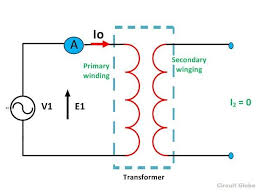The image above represents ballast circuit output voltage under no condition.

To compute for ballast circuit output voltage under no condition, three essential parameters are needed and these parameters are Resistance of the Unstrained Gauge (Rg), Ballast Resistance (Rband Input Supply Voltage (vi).

The formula for calculating ballast circuit output voltage under no condition:

vo = (Rg / Rg + Rb)vi

Where:

vo = Ballast Circuit Output Voltage
Rg = Resistance of the Unstrained gauge
Rb = Ballast Resistance
vi = Input Supply Voltage

Let’s solve an example;
Find the ballast circuit output voltage when the resistance of the unstrained guage is 12, the ballast resistance is 10 and the input supply voltage is 8.

This implies that;

Rg = Resistance of the Unstrained gauge = 12
Rb = Ballast Resistance = 10
vi = Input Supply Voltage = 8

vo = (Rg / Rg + Rb)vi
vo = (12 / 12 + 10) x 8
vo = (12 / 22) x 8
vo = 0.54 x 8
vo = 4.36

Therefore, the ballast circuit output voltage is 4.36 V.

## How to Calculate and Solve for Resistance of a Strain Guage Wire | Instrumentation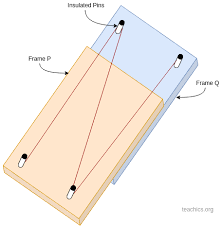The image above represents resistance of a strain guage wire.

To compute for resistance of a strain guage wire, four essential parameters are needed and these parameters are Resistivity of the Strain Gauge Wire (ρ), Length of the Strain Gauge Wire (L), Wire Constant (k) and Diameter of Wire (D).

The formula for calculating resistance of a strain guage wire:

R = ρL / kD2

Where:

R = Resistance of a Strain Gauge Wire
ρ = Resistivity of the Strain Gauge Wire
L = Length of the Strain Gauge Wire
k = Wire Constant
D = Diameter of Wire

Let’s solve an example;
Find the resistance of a strain guage wire when the resistivity of the strain guage wire is 8, the length of the strain guage wire is 2, the wire constant is 4 and the diameter of wire is 6.

This implies that;

ρ = Resistivity of the Strain Gauge Wire = 8
L = Length of the Strain Gauge Wire = 2
k = Wire Constant = 4
D = Diameter of Wire = 6

R = ρL / kD2
R = 8 x 2 / 4 x 62
R = 16 / 4 x 36
R = 16 / 144
R = 0.11

Therefore, the resistance of the strain guage wire is 0.11 Ω.

Calculating for the Resistivity of the Strain Guage Wire when the Resistance of a Strain Guage Wire, the Length of the Strain Guage Wire, the Wire Constant and the Diameter of Wire is Given.

ρ = R x kD2

Where;

ρ = Resistivity of the Strain Gauge Wire
R = Resistance of a Strain Gauge Wire
L = Length of the Strain Gauge Wire
k = Wire Constant
D = Diameter of Wire

Let’s solve an example;
Find the resistivity of the strain guage wire when the resistance of a strain guage wire is 12, the length of the strain guage wire is 8, the wire constant is 10 and the diameter of wire is 4.

This implies that;

R = Resistance of a Strain Gauge Wire = 12
L = Length of the Strain Gauge Wire = 8
k = Wire Constant = 10
D = Diameter of Wire = 4

ρ = R x kD2
ρ = 12 x (10)42
ρ = 12 x 160
ρ = 1920

Therefore, the resistivity of the strain guage wire is 1920.
Continue reading How to Calculate and Solve for Resistance of a Strain Guage Wire | Instrumentation

## How to Calculate and Solve for Strain Guage Factor | Instrumentation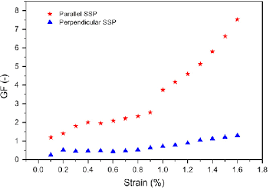The image above represents strain guage factor.

To compute for strain guage factor, four essential parameters are needed and these parameters are Change in Resistance of the Strain Gauge (ΔR), Resistance of the Strain Gauge (R), Change in Length of the Strain Gauge (ΔL) and Initial Length of the Strain Gauge (L).

The formula for calculating strain guage factor:

GF = LΔR / RΔL

Where:

GF = Strain Gauge Factor
ΔR = Change in Resistance of the Strain gauge
R = Resistance of the Strain Gauge
ΔL = Change in Length of the Strain Gauge
L = Initial length of the strain Gauge

Let’s solve an example;
Find the strain guage factor when the change in resistance of the strain guage is 12, the resistance of the strain guage is 14, the change in length of the strain guage is 16 and the initial length of the strain guage is 10.

This implies that;

ΔR = Change in Resistance of the Strain gauge = 12
R = Resistance of the Strain Gauge = 14
ΔL = Change in Length of the Strain Gauge = 16
L = Initial length of the strain Gauge = 10

GF = LΔR / RΔL
GF = 10 x 12 / 14 x 16
GF = 120 / 224
GF = 0.535

Therefore, the strain guage factor is 0.535.

Calculating for Change in Resistance of the Strain Guage when the Strain Guage Factor, the Resistance of the Strain Guage, the Change in Length of the Strain Guage and the Initial Length of the Strain Guage is Given.

ΔR = GF x RΔL / L

Where;

ΔR = Change in Resistance of the Strain gauge
GF = Strain Gauge Factor
R = Resistance of the Strain Gauge
ΔL = Change in Length of the Strain Gauge
L = Initial length of the strain Gauge

Let’s solve an example;
Find the change in resistance of the strain guage when the strain guage factor is 20, the resistance of the strain guage is 18, the change in length of the strain guage is 14 and the initial length of the strain guage is 10.

This implies that;

GF = Strain Gauge Factor = 20
R = Resistance of the Strain Gauge = 18
ΔL = Change in Length of the Strain Gauge = 14
L = Initial length of the strain Gauge = 10

ΔR = GF x RΔL / L
ΔR = 20 x (18)(14) / 10
ΔR = 20 x 252 / 10
ΔR = 5040 / 10
ΔR = 504

Therefore, the change in resistance of the strain guage is 504.